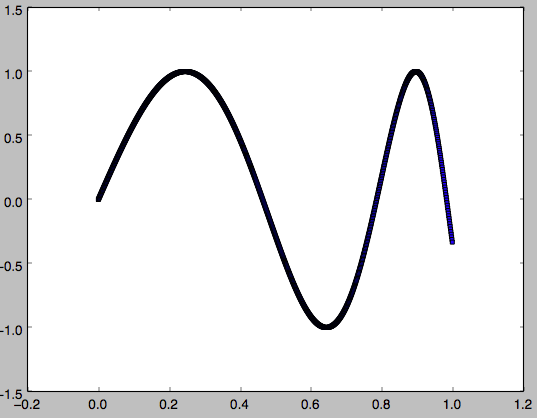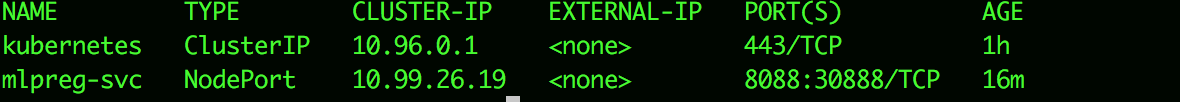# Automating the training and deployment of ML models on Kubernates

With the rise of Machine Learning and models, the need for automating and streamlining model deployment become a necessity. Pushed mostly by the fact that ML models as a new way of programming, are no longer an experimental concept but rather a day to day artifacts that can also follow a release and versioning process.

here is a link to the code used below: github

throughout this example I will :

• train a model.
• serialize it and save it.
• build a docker image with front-end web server.
• make a deployment on a kubernates cluster.

requirements:

scikit

docker

minikube & Kubernates

### Building The Model

Training  data :

our training data is going to be generated with a math function y= sin(2*π*tan(x)), where is between 0 and 1 with an increment of 0.001.

`x = np.arange(0.0, 1, 0.001).reshape(-1, 1)`

x = [[ 0. ]
[ 0.001]
[ 0.002]

………

[ 0.997]
[ 0.998]
[ 0.999]]

`y = np.sin(2 * np.pi * np.tan(x).ravel()) #with max/min values of 1,-1`Fitting the Model :

In this example, I will use  a MultiLayer Perceptron implemented by SCIKIT python library

This is what the regressor function will all the parameters ( already tuned ) :

```reg = MLPRegressor(hidden_layer_sizes=(500,), activation='relu', solver='adam', alpha=0.001,batch_size='auto',

learning_rate='constant', learning_rate_init=0.01, power_t=0.5, max_iter=1000, shuffle=True,

random_state=9, tol=0.0001, verbose=False, warm_start=False, momentum=0.9,

nesterovs_momentum=True, early_stopping=False, validation_fraction=0.1, beta_1=0.9, beta_2=0.999,

epsilon=1e-08)```
Test Data :
for testing we will use a generated set of data as well :
`test_x = np.arange(0.0, 1, 0.05).reshape(-1, 1)`
Prediction :
`test_y = reg.predict(test_x)`
Results :
continuous blue is the real output
dotted red is the predicted outputSaving the model :
I used Python object serialization framework Pickle :
`joblib.dump(reg, 'mlpreg.pkl')`
this will save your model to a file named: mlpreg.pkl

### Deploying the model

building an image :
I have created a docker image for deploying the model on a web server :

[code] FROM python:2.7.15-stretch

COPY MLPReg.py .

COPY server.py .

RUN python -m pip install –user numpy scipy matplotlib ipython jupyter pandas sympy nose

RUN python -m pip install -U scikit-learn

RUN python MLPReg.py

EXPOSE 8088

CMD python server.py

[/code]

to build the image you can run this :
`docker build -t mbenachour/mlpreg:latest .`
kubernates deployment :
this the kubernates yaml file that describes the deployment :

[code ]

apiVersion: apps/v1beta2
kind: Deployment
name: mlpreg-deployment
labels:
app: mlpreg
spec:
replicas: 3
selector:
matchLabels:
app: mlpreg
template:
labels:
app: mlpreg
spec:
terminationGracePeriodSeconds: 30
containers:
– name: mlpreg
image: mbenachour/mlpreg:latest
imagePullPolicy: “Always”
ports:
– containerPort: 8088

apiVersion: v1
kind: Service
name: mlpreg-svc
labels:
app: mlpreg
#tier: frontend
spec:
type: NodePort
ports:
– port: 8088
selector:
app: mlpreg
#tier: frontend

[/code]

you can deploy the kubernates model :
`kubectl apply -f mlp.yml`
to check on the status of your kubernates services :
`kubectl get services`
you should see something similar to this :### Making predictions

to get the kubernates cluster IP address, in my case I’m using minikube the command line :

```\$minikube service mlpreg-svc --url

http://192.168.99.105:32397```
to make a prediction using the API for an input of 0.1### Pipeline.ai

a lot of products have been introduced to help solve this problem, one of them is Chris Fregly project: pipeline.ai
the project gives you the possibility to create-train-deploy models using different frameworks :
– tensorflow
– scikit
– pytorch
implementing a lot of ML most used algorithms like linear regression.